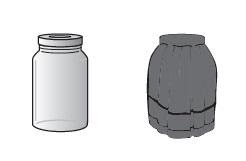Courses
Courses for Kids
Free study material
Offline Centres
MoreLast updated date: 29th Nov 2023
Total views: 279.9k
Views today: 2.79k

# The rate of photosynthesis in pond water can be calculated a) By subtracting the level in the covered jar from the level in the original sample and adding that to the number to the level in the uncovered jar.b) By adding the level in the covered jar to the level in the original sample and subtracting that from the number to the level in the uncovered jar. c) By subtracting the level in the uncovered jar from the level in the original sample and adding that to the number to the level in the covered jar.d) By adding the level in the uncovered jar to the level in the original sample and subtracting that from the number to the level in the covered jar.e) By adding the level in the covered jar to the level in the uncovered jar and subtracting that number from the level in the original sample.Verified
279.9k+ views
Hint: The rate of photosynthesis is a gross measure of the rate at which a plant captures radiant energy and fixes it in organic carbon compounds. The rate of photosynthesis is affected by both internal plant factors and external factors. Number, scale, and orientation of leaves mesophyll cells and chloroplast, internal $C{O_2}$concentration, and chlorophyll amount are among the plant factors. Light, $C{O_2}$, temperature, ${H_2}O$, and other external factors.

This is the correct method of calculating the rate of photosynthesis.
Option (a) is correct.
We will get the incorrect results by adding the level in the covered jar to the level in the original sample and subtracting that from the number to the level in the uncovered jar.
Option (b) is incorrect.
By subtracting the level in the uncovered jar from the level in the original sample and adding that to the number to the level in the covered jar will give wrong results.
Option (c) is incorrect.
We cannot find the rate of photosynthesis using this way.
Option (d) is incorrect.
The correct answer cannot be obtained by subtracting the combined level in a covered and uncovered jar from the original sample.
Option (e) is incorrect.

So, the correct answer is option (a).

Note:
The common method used to measure the rate of oxygen evolution during photosynthesis is by noting the volume changes of the bubbles that evolved. When the light is closer to the beaker, more oxygen bubbles are formed. As a result, a higher light intensity leads to a faster rate of photosynthesis.
$C{O_2} + {H_2}O\xrightarrow{{light + chlorophyll}}Glu\cos e + {O_2}$

$C{O_2}$: It is the limiting factor for photosynthesis because it is found in very less amount in atmosphere. The saturation & compensation point for ${C_3}$ and ${C_4}$ plants is $450ppm$ & $10 - 18ppm$ and $360ppm$ & $10ppm$, respectively.
Temperature: ${C_4}$ plants respond to high temperature while ${C_3}$ plants have lower optimum temperature.
Water: It indirectly affects photosynthesis. Water stress causes stomata to close which stops $C{O_2}$ entry and thereby decrease in the rate of photosynthesis.